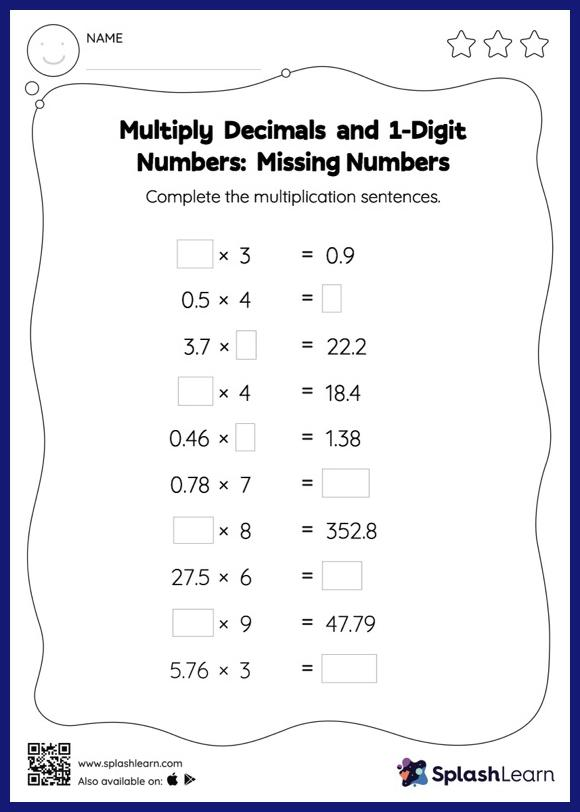# Multiply Decimals and 1-Digit Numbers: Missing Numbers Worksheet

Home > Multiply Decimals and 1-Digit Numbers: Missing NumbersThis worksheet gives students an opportunity to apply the concepts of multiplication to multiply decimals and 1-digit numbers. Students may omit the decimal point when multiplying a decimal by a one-digit number and then add it when writing the answer. Students find missing numbers in multiply decimals and 1-digit numbers worksheet using this concept.